# 基于 HTML5 WebGL 智能城市的模拟运行

2019/05/05 07:56

## 前言## 代码实现

let dm = this.dm = new ht.DataModel();
let entryG3d = this.entryG3d = new ht.graph3d.Graph3dView(dm);
entryG3d.addToDOM(); // 将场景添加到页面中

HT 的组件一般都会嵌入 BorderPane、SplitView 和 TabView 等容器中使用，而最外层的 HT 组件则需要用户手工将 getView() 返回的底层 div 元素添加到页面的 DOM 元素中，这里需要注意的是，当父容器大小变化时，如果父容器是 BorderPane 和 SplitView 等这些 HT 预定义的容器组件，则 HT 的容器会自动递归调用孩子组件 invalidate 函数通知更新。但如果父容器是原生的 html 元素， 则 HT 组件无法获知需要更新，因此最外层的 HT 组件一般需要监听 window的窗口大小变化事件，调用最外层组件 invalidate 函数进行更新。

addToDOM = function(){
var self = this,
view = self.getView(), // 获取组件 div
style = view.style;
document.body.appendChild(view); // 将组件添加到文档对象中
style.left = '0';
style.right = '0';
style.top = '0';
style.bottom = '0';
window.addEventListener('resize', function () { self.iv(); }, false); // 监听窗口变化，刷新组件
}

接下来反序列化城市场景 json。

ht.Default.xhrLoad('scenes/园区/城市demo.json', (text) => {
let json = ht.Default.parse(text); // 还原 json 字符串
let scene = json.scene; // 获取 json 中设置的投影参数
entryG3d.setEye(scene.eye); // 设置视角
entryG3d.setCenter(scene.center); // 设置目标中心点
entryG3d.setFar(scene.far); // 设置远端截面位置
entryG3d.setNear(scene.near); // 设置近端截面位置
dm.deserialize(text); // 场景反序列化
this.setSkyBox(entryG3d); // 设置天空球
this.initentryG3dEvent(); // 添加场景监听事件
this.startAnimate(); // 启动城市动画
this.startCarAnimate(['car1Line'], dm.getDataByTag('car1')); // 启动消防小车1动画
this.startCarAnimate(['car2Line'], dm.getDataByTag('car2')); // 启动消防小车2动画
});

1. 环境光贴图：将贴图影像渲染在场景中，通过 node.s('envmap', 0.5) 来设置节点的渲染程度，主要用于地板添加物体倒影效果，也可像本 demo 一样添加星光点缀。

dataModel.setEnvmap('环境光贴图.png'); // 贴图要求宽高像素为 2^n
node.s('envmap', 0.1);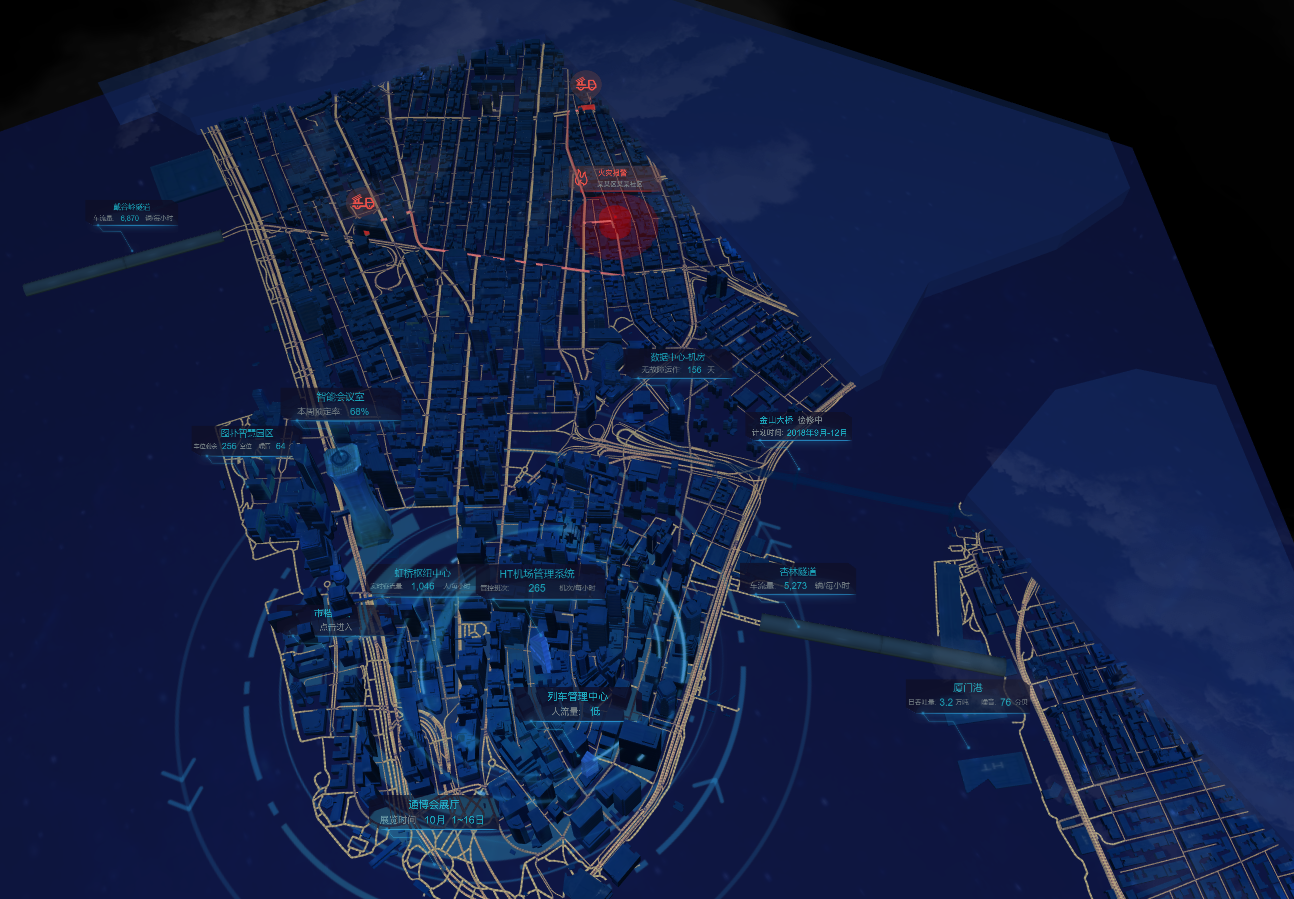2. 辉光：本是指低压气体中气体放电的物理现象，用在 3D 场景中将亮色突出显示，呈现一种发光的效果，可控制辉光强度，辉光显示的范围和发光阀值。

g3d.enablePostProcessing('Bloom', true); // 开启辉光
module = g3d.getPostProcessingModule('Bloom');
module.strength = 0.18; // 强度
module.threshold = 0.62; // 阈值
g3d.iv(); // 刷新拓扑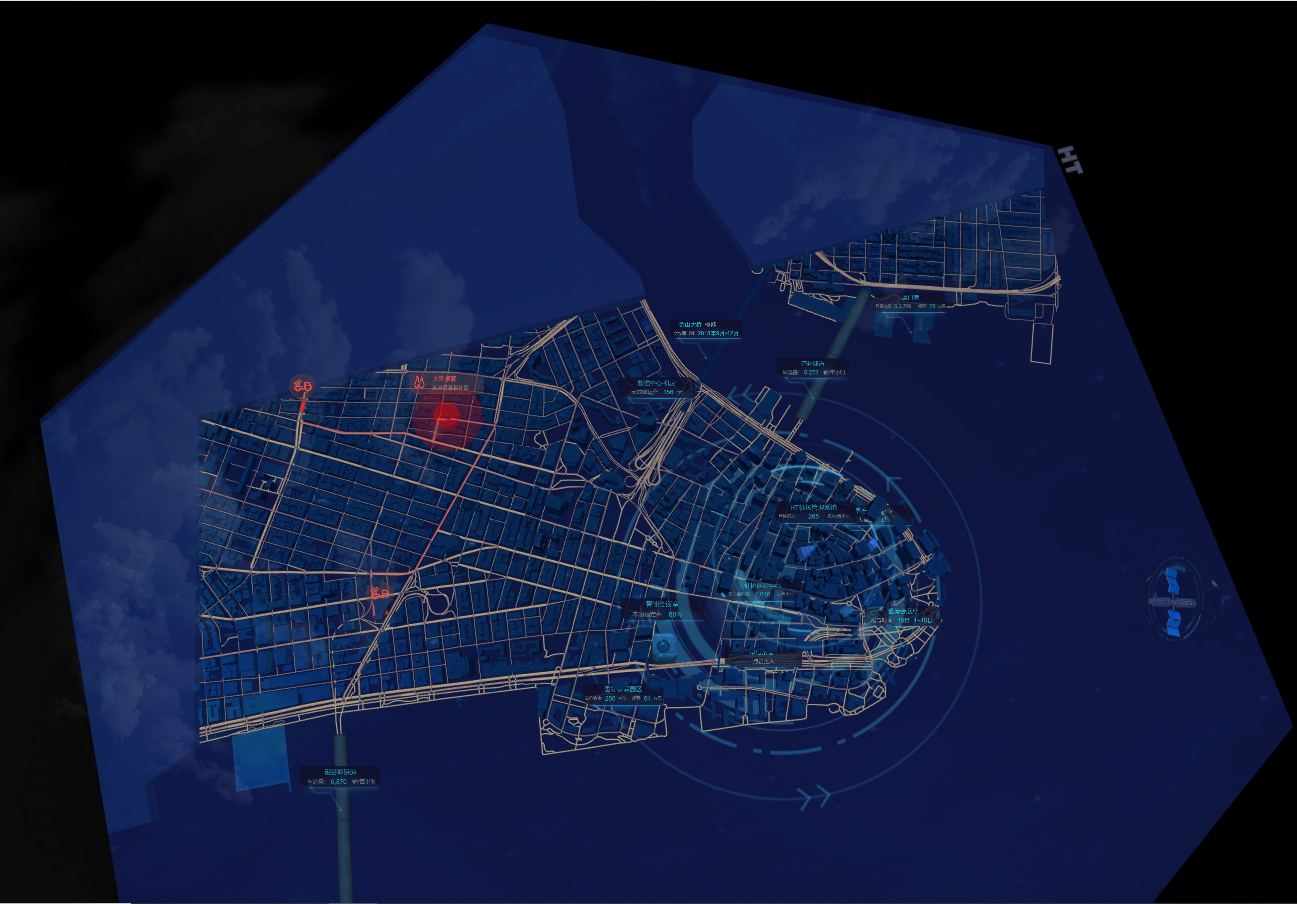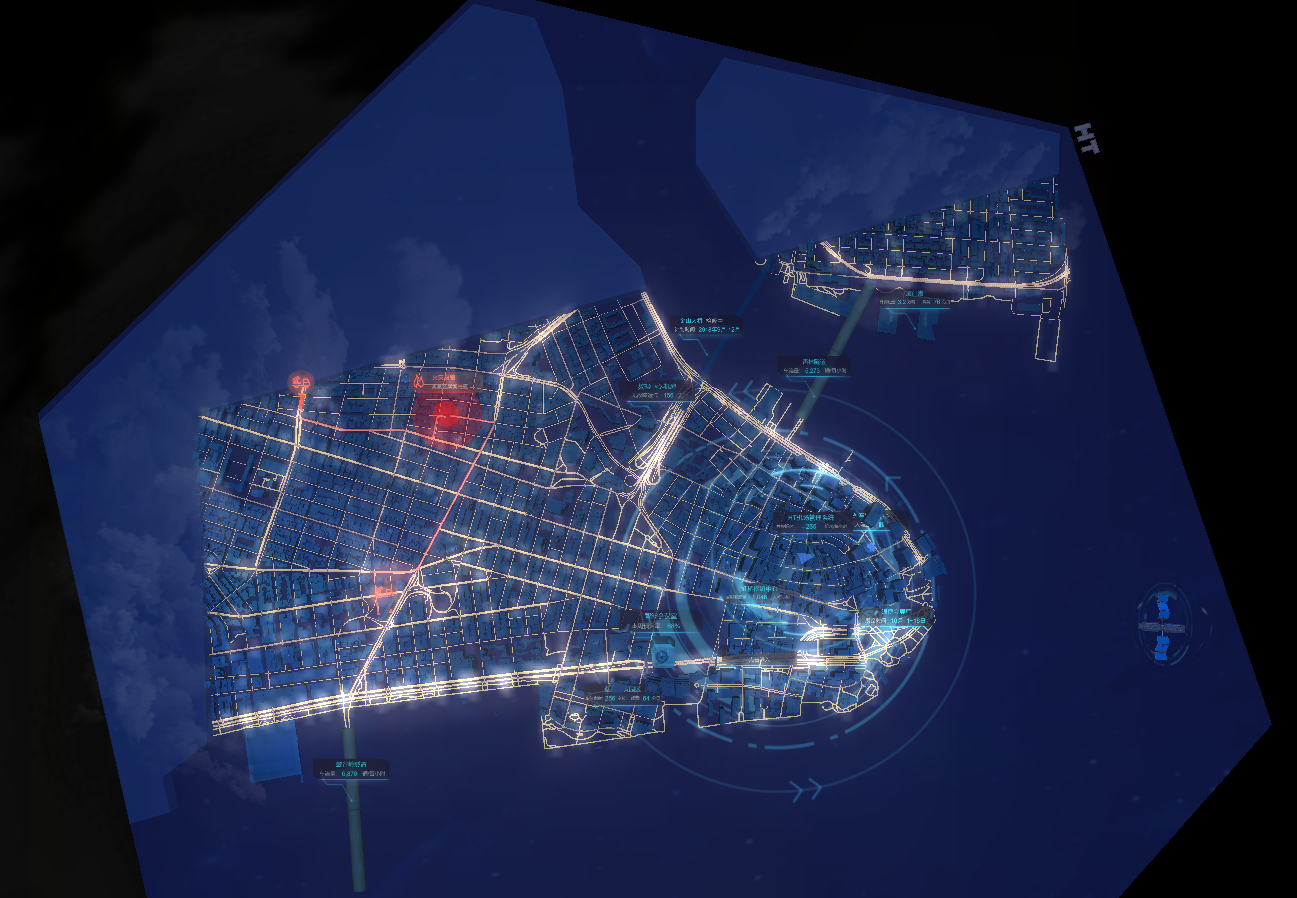3. 景深：对场景中心周围的清晰程度的控制，将周围虚化，美化画面，突出主体，增强透视，可控制景深阀值（周围模糊范围程度）。

g3d.enablePostProcessing('Dof', true); // 开启景深
module = g3d.getPostProcessingModule('Dof');
module.aperture = 0.18; // 景深阀值
module.image= '景深贴图.png'; // 景深贴图
g3d.iv(); // 刷新拓扑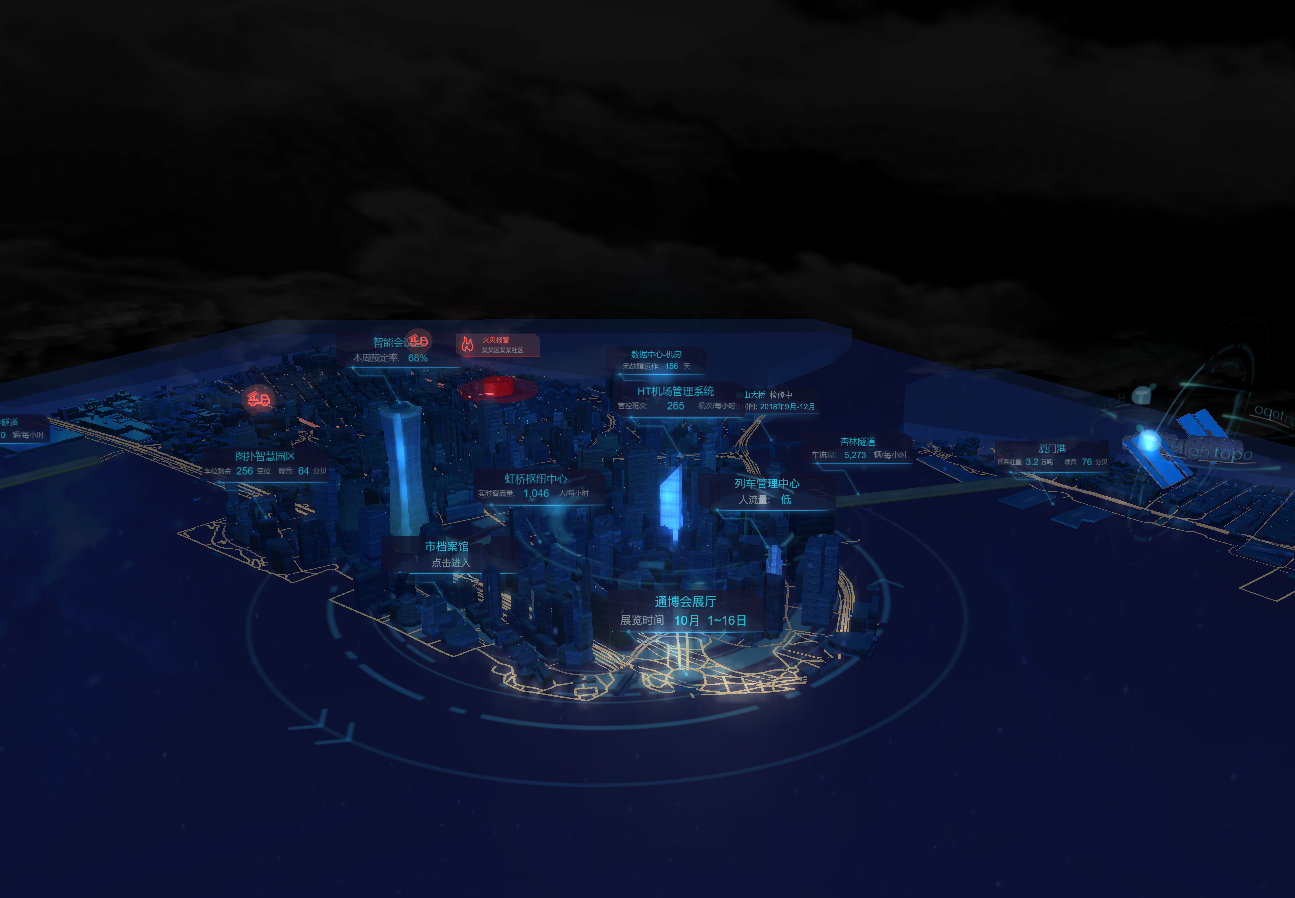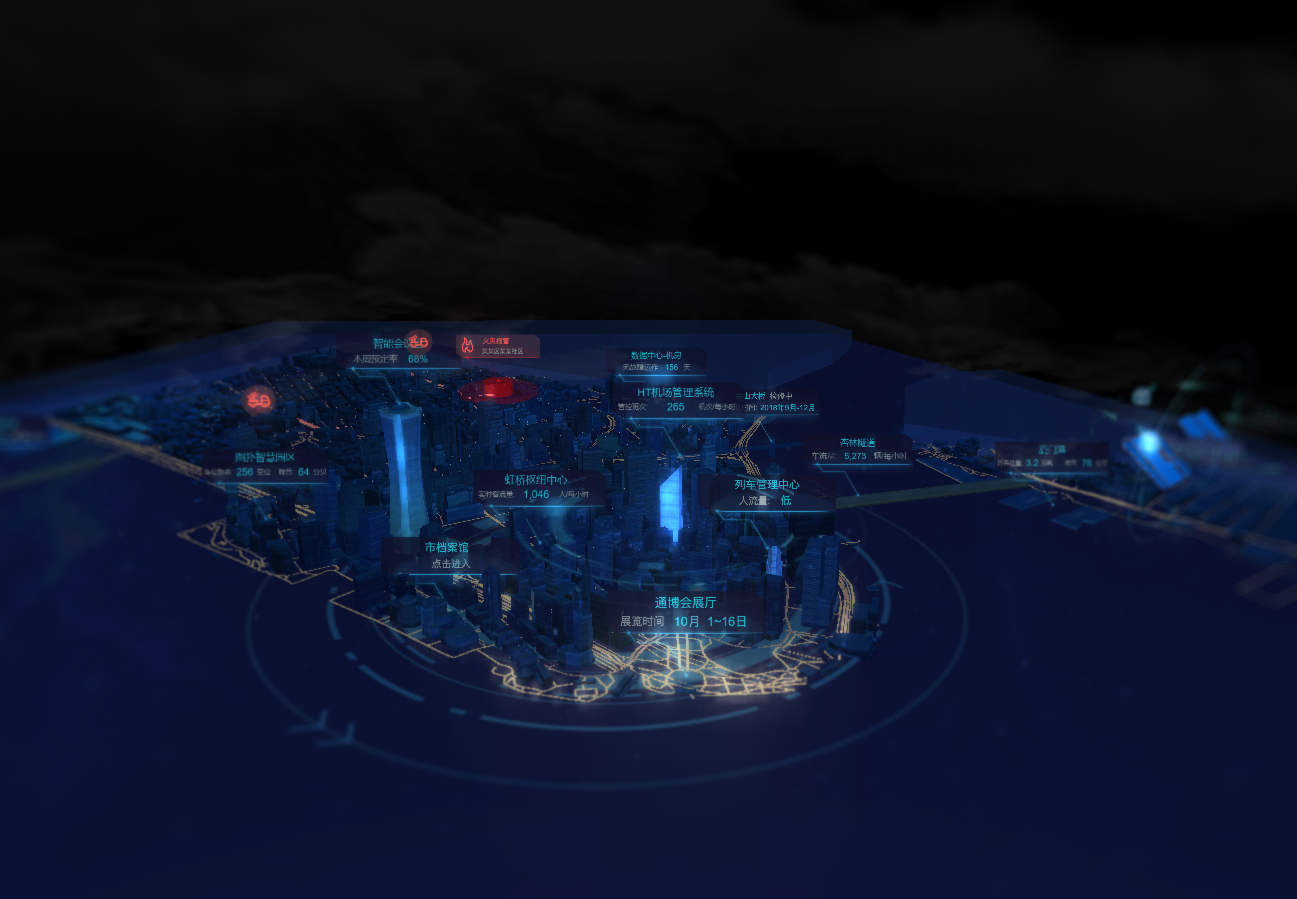4. 天空球：将场景模型放置在一个大的球体中，球体内部进行贴图，来模拟天空。

node = new ht.Node()
node.s({
'shape3d':'sphere', // 球体
'shape3d.image': 'earth' // 贴图路径
});
node.s3(10000, 10000, 10000);
g3d.setSkyBox(node); // 设置天空球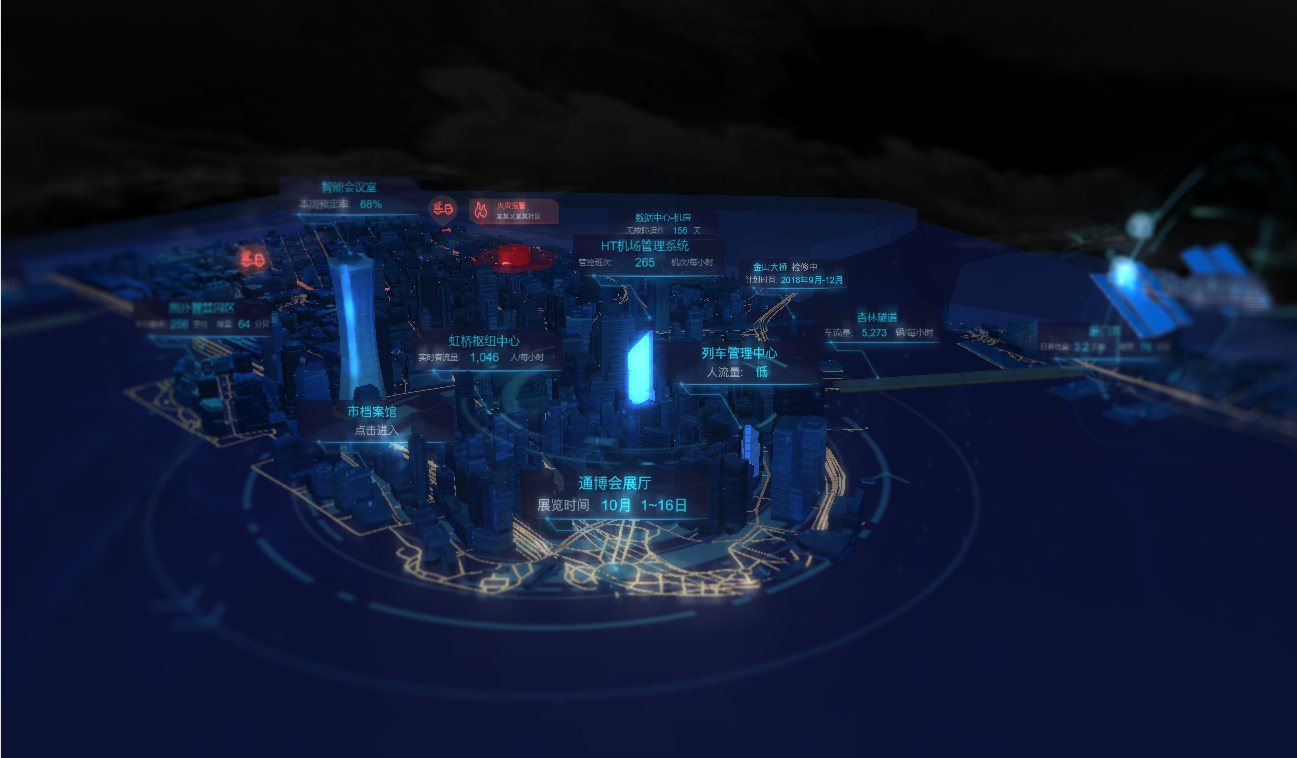HT 中调度进行的流程是，先通过 dataModel 添加调度任务，dataModel 会在调度任务指定的时间间隔到达时， 遍历 dataModel 所有图元回调调度任务的 action 函数，可在该函数中对传入的 data 图元做相应的属性修改以达到动画效果。

dataModel.addScheduleTask(task) 添加调度任务，其中 task 为 json 对象，可指定如下属性：

• interval：间隔毫秒数，默认值为10
• enabled：是否启用开关，默认为 true
• action：间隔动作函数，该函数必须设置

startAnimate() {
let dr = Math.PI / 180 * 2, PI2 = Math.PI * 2;
let dm = this.dm;
let rotateOval = dm.getDataByTag('rotateOval'); // 获取城市列车模型
let logo = dm.getDataByTag('logo'); // 获取城市卫星模型

// 设置动画参数
interval: 100,
action: function(data){
if(data === rotateOval || data === logo){
data.setRotation((data.getRotation() - dr) % PI2);
}
}
};

// 获取建筑物数据面板
let dglsd = dm.getDataByTag('dglsd'); // 戴谷岭隧道
let xlsd = dm.getDataByTag('xlsd'); // 杏林隧道
let xmg = dm.getDataByTag('xmg'); // 厦门港
let jcglzx = dm.getDataByTag('jcglzx'); // 机场管理中心

// 模拟建筑物数据面板的动态展示
interval: 1000,
action: function(data){
if(data === dglsd){
data.a('carHour', util.randomNumBetween(3000, 6000));
}
if(data === xlsd){
data.a('carHour', util.randomNumBetween(3000, 6000));
}
if(data === xmg){
data.a('ttl', util.randomNumBetween(3, 10));
data.a('zy', util.randomNumBetween(0, 100));
}
if(data === jcglzx){
data.a('sskll', util.randomNumBetween(500, 2000));
}
}
};
}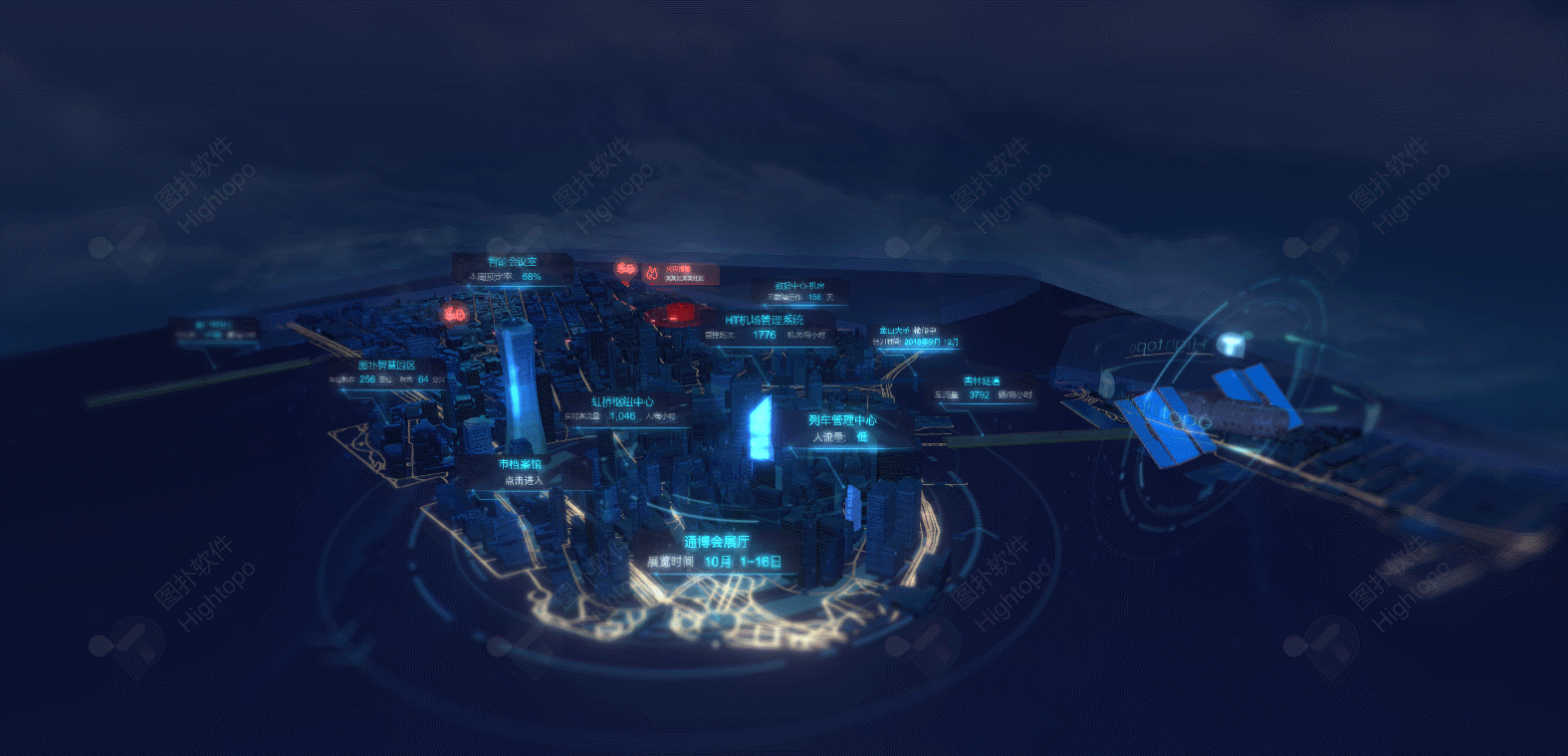demo 还模拟了消防车赶往火灾发生地，动画如下：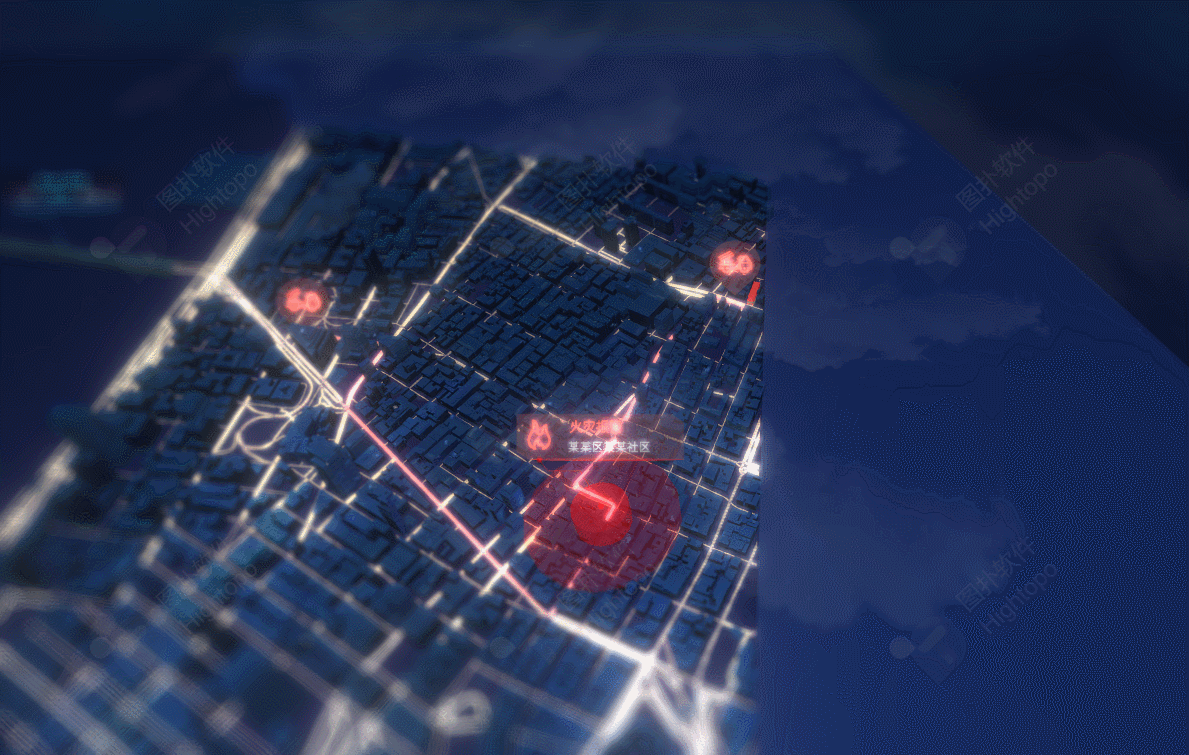ht.Default.startAnim({
frames: 12, // 动画帧数
interval: 10, // 动画帧间隔毫秒数
easing: function(t){ return t * t; }, // 动画缓动函数，默认采用 ht.Default.animEasing
finishFunc: function(){ console.log('Done!') }, // 动画结束后调用的函数。
action: function(v, t){ // action函数必须提供，实现动画过程中的属性变化。
node.setPosition( // 此例子展示将节点node从位置p1动画到位置p2。
p1.x + (p2.x - p1.x) * v,
p1.y + (p2.y - p1.y) * v
);
}
});

ht.Default.startAnim({
duration: 500, // 动画周期毫秒数，默认采用ht.Default.animDuration
action: function(v, t){
...
}
});

startCarAnimate(polylineTagArr, airNode) { // 传入消防车行驶路线 tag(唯一标签)组和消防车模型节点
let dm = this.dm;
let entryG3d = this.entryG3d;
let curIndex = 0;
let polyline = dm.getDataByTag(polylineTagArr[curIndex]); // 获取消防路线 ht.PolyLine（http://www.hightopo.com/guide/guide/core/shape/ht-shape-guide.html#ref_different）
let prePos = airNode.p3(); // 获取节点初始位置
let preRotate = airNode.r3(); // 获取节点初始旋转角度
let lineLength = entryG3d.getLineLength(polyline); // 获取管道长度
let params = { // 设置消防车行驶动画参数
duration: 10000,
easing: function(t){
return t * t;
},
action: function(v, t){
let offset = entryG3d.getLineOffset(polyline, lineLength * v), // 获取管道指定比例的偏移信息
point = offset.point,
px = point.x,
py = point.y,
pz = point.z,
tangent = offset.tangent,
tx = tangent.x,
ty = tangent.y,
tz = tangent.z;
airNode.p3(px, py, pz); // 移动消防车到下一位置
airNode.lookAt([px + tx, py + ty, pz + tz], 'front'); // 消防车沿着管道方向转向
},
finishFunc: function(){
if(curIndex < polylineTagArr.length - 1) { // 进入下一段路线
curIndex++;
}
else {
curIndex = 0; // 返回第一段路线
}
if(curIndex === 0) { // 消防车返回原点
airNode.p3(prePos);
airNode.r3(preRotate);
}
polyline = dm.getDataByTag(polylineTagArr[curIndex]);
lineLength = entryG3d.getLineLength(polyline);
this.carAni = ht.Default.startAnim(params); // 执行下一段行驶动画
}
};
this.carAni = ht.Default.startAnim(params);
}

1. g3d.getLineLength(edgeOrPolyLine)

根据参数 edgeOrPolyLine 方法命名我们就可以知道此方法是获取连线或管道的长度。

2. g3d.getLineOffset(edgeOrPolyLine, offset)

• edgeOrPolyLine  连线或者管道
• offset 偏移百分比

此方法获取连线或者管道的偏移信息，返回 { point, tangent }，其中 point 是 3D 坐标，tangent 是 point 点沿当前线的切线向量。

3. node.lookAt(point, direction)

• point 3D 坐标点
• direction 节点某一面，值可为 ‘front | back | left  | right | top | bottom’

将节点的某一面朝向空间某坐标点。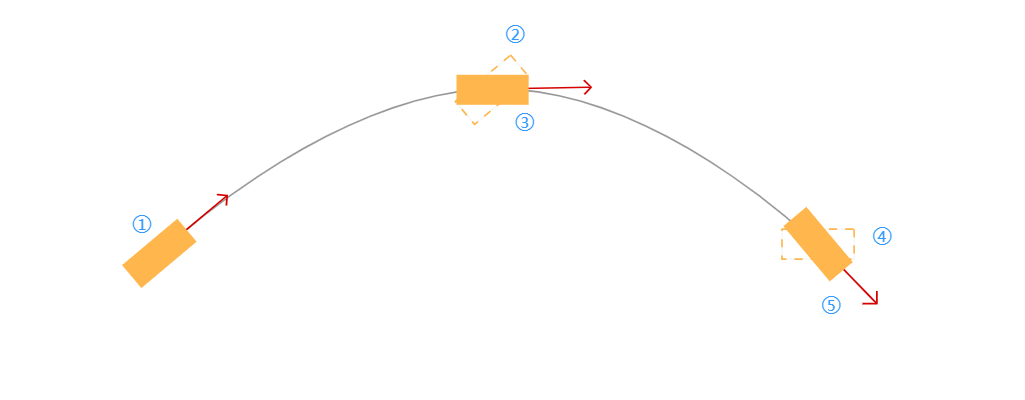1. 起始位置；

2. 通过 setPosition3d(point.x, point.y, point.z) 到达下一位置，红线为 tangent 切线向量；

3. 通过 node.lookAt([point.x + tangent.x, point.y + tangent.y, point.z + tangent.z], 'front'); 将车头摆向切线方向；

4.5. 重复以上步骤。

其中 [point.x + tangent.x, point.y + tangent.y, point.z + tangent.z] 即为目标点 target 的坐标。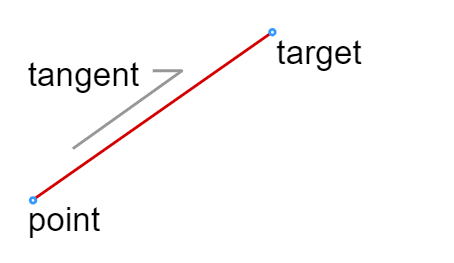## 总结

智能城市系统还包括智能楼宇的管理：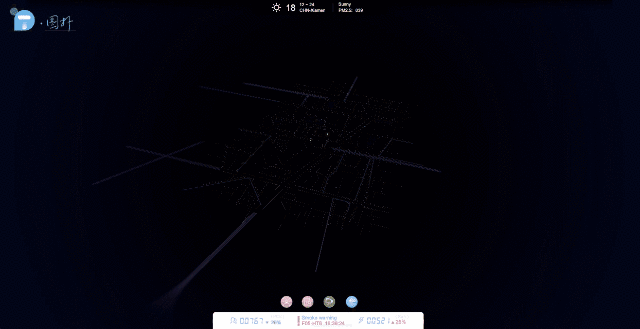http://www.hightopo.com/demo/ht-smart-building/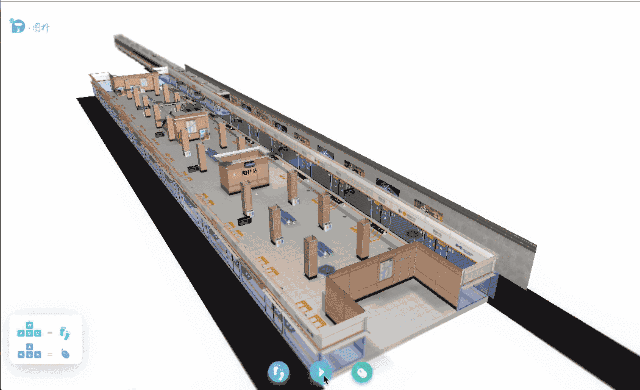http://www.hightopo.com/demo/ht-subway/### 作者的其它热门文章

0
1 收藏

0 评论
1 收藏
0